Next: Surface Tension Up: Waves in Incompressible Fluids Previous: Gravity Waves at an

# Steady Flow over a Corrugated Bottom

Consider a stream of water of mean depth, and uniform horizontal velocity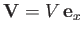, that flows over a corrugated bottom whose elevation is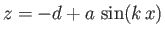, where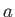is much smaller than. Let the elevation of the free surface of the water be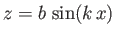. We wish to determine the relationship betweenand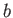.

We expect the velocity potential, perturbed pressure, and vertical displacement of the water to be of the form (11.91), (11.92), and (11.93), respectively, with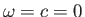, because we are looking for a stationary (i.e., non-propagating) perturbation driven by the static corrugations in the bottom. The boundary condition at the bottom is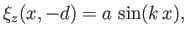(11.104)

which yields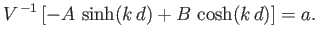(11.105)

At the free surface, we have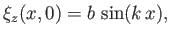(11.106)

which gives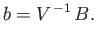(11.107)

In addition, pressure balance across the free surface yields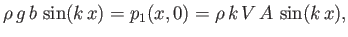(11.108)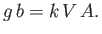(11.109)

Hence, from Equations (11.105), (11.107), and (11.109),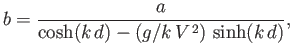(11.110)

or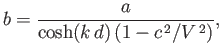(11.111)

where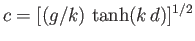is the phase velocity of a gravity wave of wave number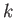. [See Equation (11.21).] It follows that the peaks and troughs of the free surface coincide with those of the bottom when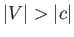, and the troughs coincide with the peaks, and vice versa, when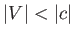. If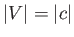then the ratio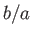becomes infinite, implying that the oscillations driven by the corrugations are not of small amplitude, and, therefore, cannot be described by linear theory.Next: Surface Tension Up: Waves in Incompressible Fluids Previous: Gravity Waves at an
Richard Fitzpatrick 2016-03-31